# PSAT Math : How to find the degree of a polynomial

## Example Questions

### Example Question #1 : How To Find The Degree Of A Polynomial

Give the degree of the polynomial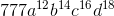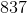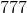Explanation:

The polynomial has one term, so its degree is the sum of the exponents of the variables: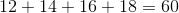### Example Question #2 : How To Find The Degree Of A Polynomial

Give the degree of the polynomial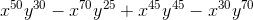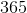Explanation:

The degree of a polynomial in more than one variable is the greatest degree of any of the terms; the degree of a term is the sum of the exponents. The degrees of the terms in the given polynomial are: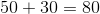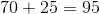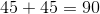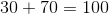The degree of the polynomial is the greatest of these degrees, 100.

### Example Question #3 : How To Find The Degree Of A Polynomial

Give the degree of the polynomial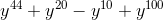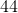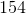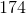Explanation:

The degree of a polynomial in one variable is the greatest exponent of any of the powers of the variable. The terms have as their exponents, in order, 44, 20, 10, and 100; the greatest of these is 100, which is the degree.

### Example Question #4 : How To Find The Degree Of A Polynomial

Give the degree of the polynomial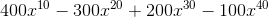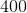Explanation:

The degree of a polynomial in one variable is the greatest exponent of any of the powers of the variable. The terms have as their exponents, in order, 10, 20, 30, and 40; 40 is the greatest of them and is the degree of the polynomial.

### Example Question #5 : How To Find The Degree Of A Polynomial

Which of these polynomials has the greatest degree?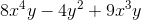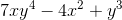All of the polynomials given in the other responses have the same degree.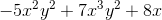All of the polynomials given in the other responses have the same degree.

Explanation:

The degree of a polynomial is the highest degree of any term; the degree of a term is the exponent of its variable or the sum of the exponents of its variables, with unwritten exponents being equal to 1. For each term in a polynomial, write the exponent or add the exponents; the greatest number is its degree. We do this with all four choices: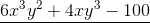: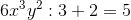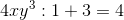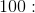A constant term has degree 0.

The degree of this polynomial is 5.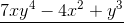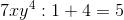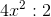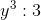The degree of this polynomial is 5.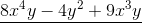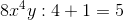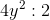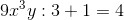The degree of this polynomial is 5.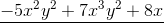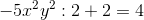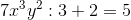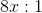The degree of this polynomial is 5.

All four polynomials have the same degree.

### Example Question #6 : How To Find The Degree Of A Polynomial

Which of the following monomials has degree 999?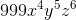None of the other responses is correct.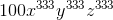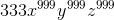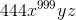Explanation:

The degree of a monomial term is the sum of the exponents of its variables, with the default being 1.

For each monomial, this sum - and the degree - is as follows: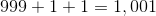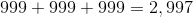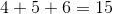(note - 999 is the coefficient)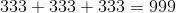is the correct choice.

### Example Question #7 : How To Find The Degree Of A Polynomial

Find the degree of the polynomial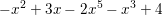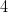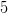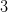Explanation:

The degree of the polynomial is the largest degree of any one of it's individual terms.The degree of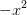is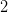The degree of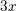isThe degree of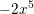isThe degree of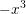isThe degree ofis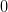is the largest degree of any one of the terms of the polynomial, and so the degree of the polynomial is.